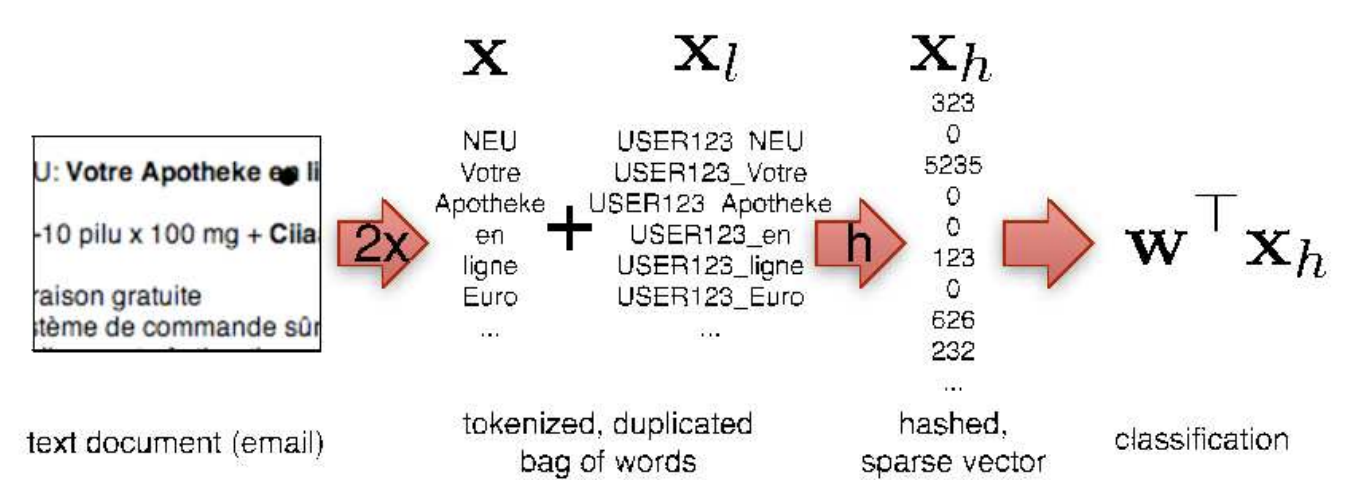# Hashing for Linear Functions

linear function
hashing
Published

March 1, 2011This is the first of a few posts on hashing. It’s an incredibly powerful technique when working with discrete objects and sequences. And it’s also idiot-proof simple. I decided to post it after listening to a talk this morning where the speaker proposed something really complicated for what should be very simple.

A hash function is (in its ideal CS version) a mapping $$h$$ from a domain $$X$$ into a set $$\{0 \ldots N-1\}$$ of integers such that $$h(x)$$ is essentially random. A fast implementation is e.g. Murmur hash. Note that as long as the hash is good it does not matter which one you use (I have received the latter question at least twice from referees in the past. It’s about as meaningful as asking which random number generator was used for sampling). It does matter if you’re designing cryptographic algorithms but for most of machine learning the quality bar is a lot lower. When we want to compute inner products of the form

$f(x) = \langle \phi(x), w \rangle = \sum_i \phi_i(x) w_i$

we might run into a big problem: whenever the feature map is too high-dimensional we will not have enough memory to store $$w$$. For instance, in the olden times (Eskin, Leslie and Noble, 2002)people would use substrings up to a certain order for protein classification. A second problem is that we might have to store a dictionary for computing the features of $$x$$, e.g. when we have a bag of words representation. It is quite likely that the dictionary will take up much more memory than the actual parameter vector $$w$$. On top of that, we need to write code to generate the dictionary. Instead of that, let’s be lazy and do the following:

$f(x) = \sum_{i: \phi(x)_i \neq 0} \phi(x)_i w_{h(i)} \sigma(i)$

Here $$h$$ is a hash function with range small enough to fit $$w$$ into memory and $$σ$$ is a binary $$\{\pm 1\}$$ hash function. This looks pretty simple. It’s essentially the sketch that Charikar, Chen and Farrach-Colton suggested in 2003 for data streams. But it’s also very powerful. In particular one can show the following:

• The inner products are unbiased relative to the original linear function.
• The variance decreases with $$1/N$$.
• No dictionary is needed. The hash function acts as our lookup device for the index. This is a massive space saver and it also is typically much faster.
• We can use this even when we don’t know the full dictionary at the time we start running an online solver. E.g. whenever we get new users we don’t need to allocate more memory for them.
• This can (relatively) easily be extended to approximate matches. Say I have the string hash. Then I can simply insert the strings *ash, h*sh, ha*h, has* to represent approximate matches.
• We can also extend it to substrings - simply add them to the hash table. This yields e.g. sub, ubs, bst, str, tri, rin, ing, ngs when we are interested in trigrams. In general for all n-grams the cost is linear in $$n$$ and the length of the document.
• Adding some weighting to the features is trivial. Just do it. Go wild. Add meaningful substrings. Personalize for users. Add tasks. Use for social networks. Use collaborative filtering (more on this later).
• To get started have a look at John Langford’s Vowpal Wabbit. The theory can be found in this AISTATS and this ICML paper. Note: the proof of the tail bound in the ICML paper is broken.